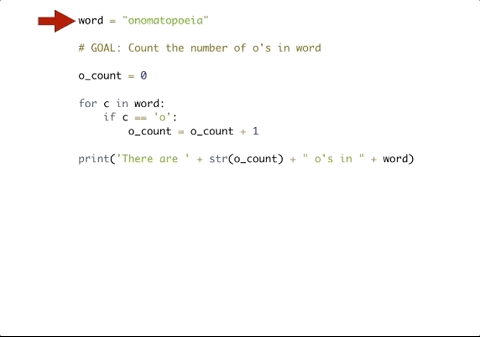# 8.10. The Accumulator Pattern with Conditionals¶

Sometimes when we’re accumulating, we don’t want to add to our accumulator every time we iterate. Consider, for example, the following program which counts the number of letters in a phrase.

Here, we initialize the accumulator variable to be zero on line two.

We iterate through the sequence (line 3).

The update step happens in two parts. First, we check to see if the value of char is not a space. If it is not a space, then we update the value of our accumulator variable tot (on line 6) by adding one to it. If that conditional proves to be False, which means that char is a space, then we don’t update tot and continue the for loop. We could have written tot = tot + 1 or tot += 1, either is fine.

At the end, we have accumulated a the total number of letters in the phrase. Without using the conditional, we would have only been able to count how many characters there are in the string and not been able to differentiate between spaces and non-spaces.

We can use conditionals to also count if particular items are in a string or list. The following code finds all occurrences of vowels in the following string.

We can also use == to execute a similar operation. Here, we’ll check to see if the character we are iterating over is an “o”. If it is an “o” then we will update our counter.## 8.10.1. Accumulating the Max Value¶

We can also use the accumulation pattern with conditionals to find the maximum or minimum value. Instead of continuing to build up the accumulator value like we have when counting or finding a sum, we can reassign the accumulator variable to a different value.

The following example shows how we can get the maximum value from a list of integers.

Here, we initialize best_num to zero, assuming that there are no negative numbers in the list.

In the for loop, we check to see if the current value of n is greater than the current value of best_num. If it is, then we want to update best_num so that it now is assigned the higher number. Otherwise, we do nothing and continue the for loop.

You may notice that the current structure could be a problem. If the numbers were all negative what would happen to our code? What if we were looking for the smallest number but we initialized best_num with zero? To get around this issue, we can initialize the accumulator variable using one of the numbers in the list.

The only thing we changed was the value of best_num on line 2 so that the value of best_num is the first element in nums, but the result is still the same!

For each string in the list words, find the number of characters in the string. If the number of characters in the string is greater than 3, add 1 to the variable num_words so that num_words should end up with the total number of words with more than 3 characters.
Challenge For each word in words, add ‘d’ to the end of the word if the word ends in “e” to make it past tense. Otherwise, add ‘ed’ to make it past tense. Save these past tense words to a list called past_tense.